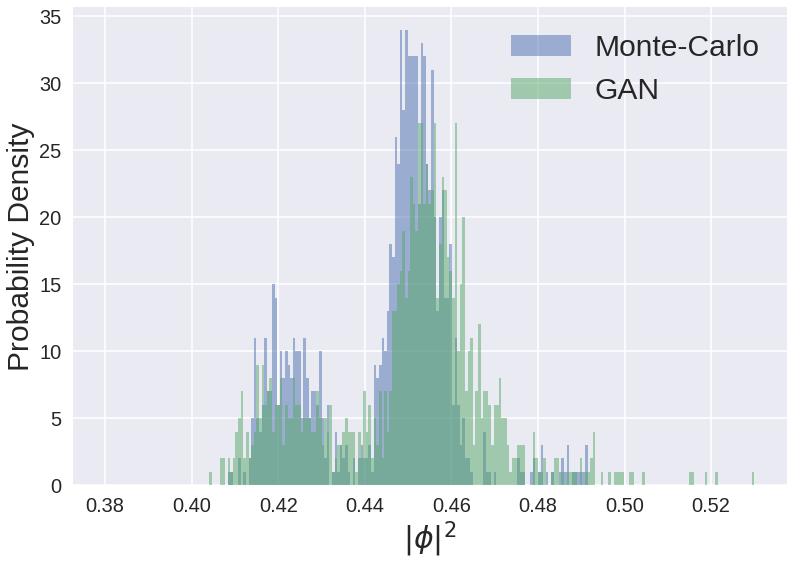• # Research

Research group of Gergely Endrődi

Gergely Endrődi

Research interests

• finite-temperature quantum field theory
• QCD phase diagram; confinement and chiral symmetry breaking
• background magnetic fields in quantum field theories
• simulation algorithms for lattice field theories
• Dirac eigenvalues on the lattice
• effective theories for QCD
• physics of compact stars

You can find my complete publication list on iNSPIREhep.

Recent research highlights

 Superconformal values of the speed of sound are reached in isospin-asymmetric QCD matter. [link]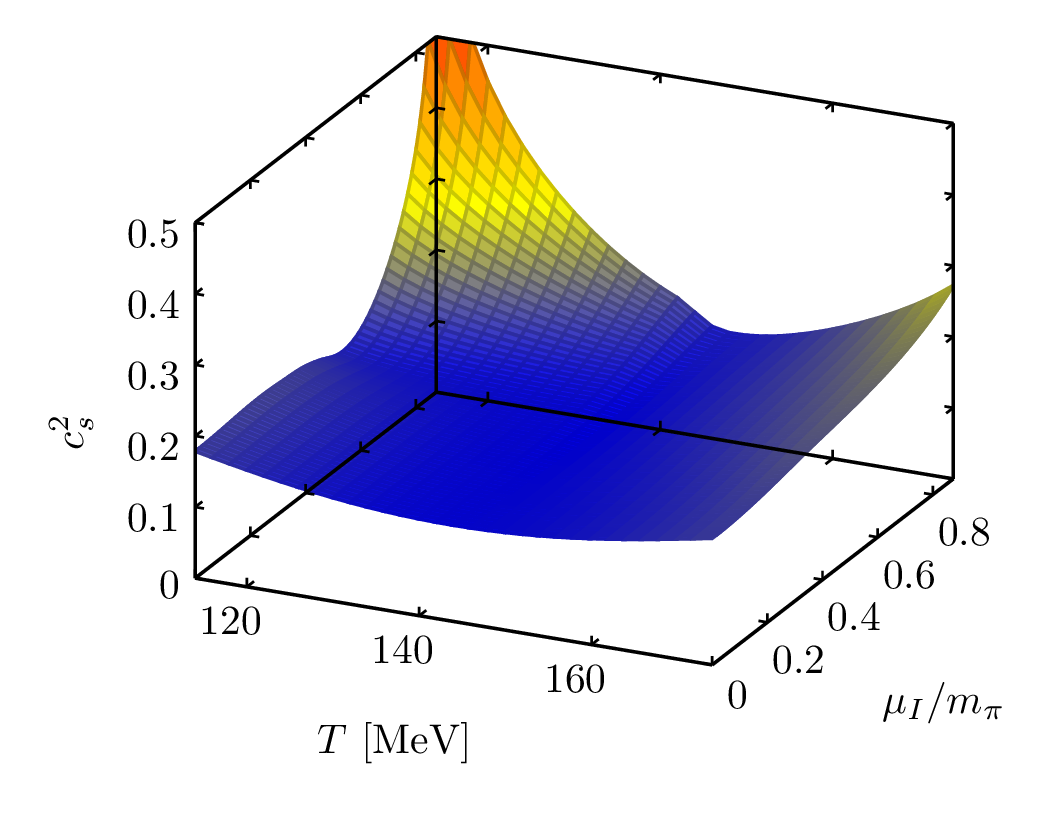Equilibrium charge distribution in the presence of an oscillatory external electric field[link]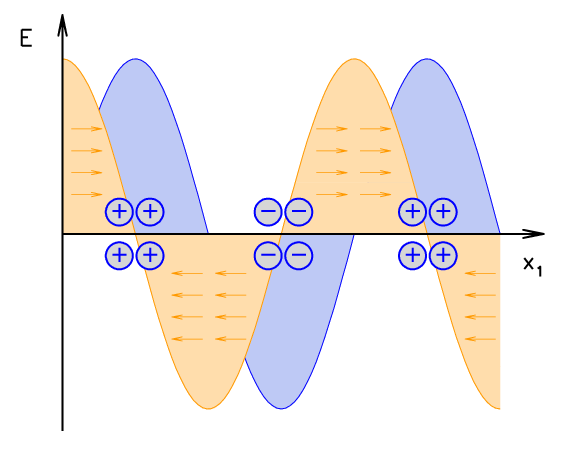Polyakov loop sectors and Roberge-Weiss phase transitions in the QCD phase diagram from perturbation theory [link]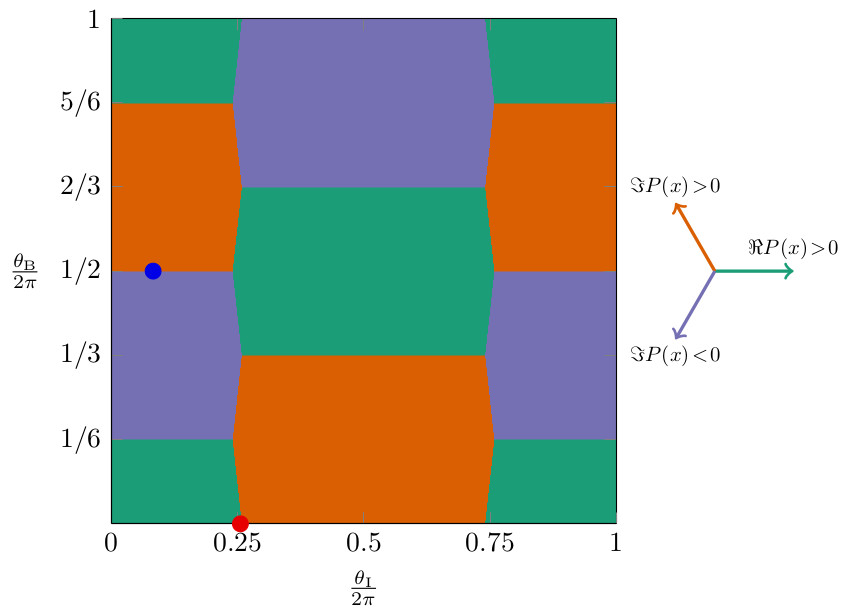Topologically nontrivial ground states emerge in the spontaneously broken phase of a spin model [link]The magnetic permeability of strongly interacting matter is calculated with a new method [link].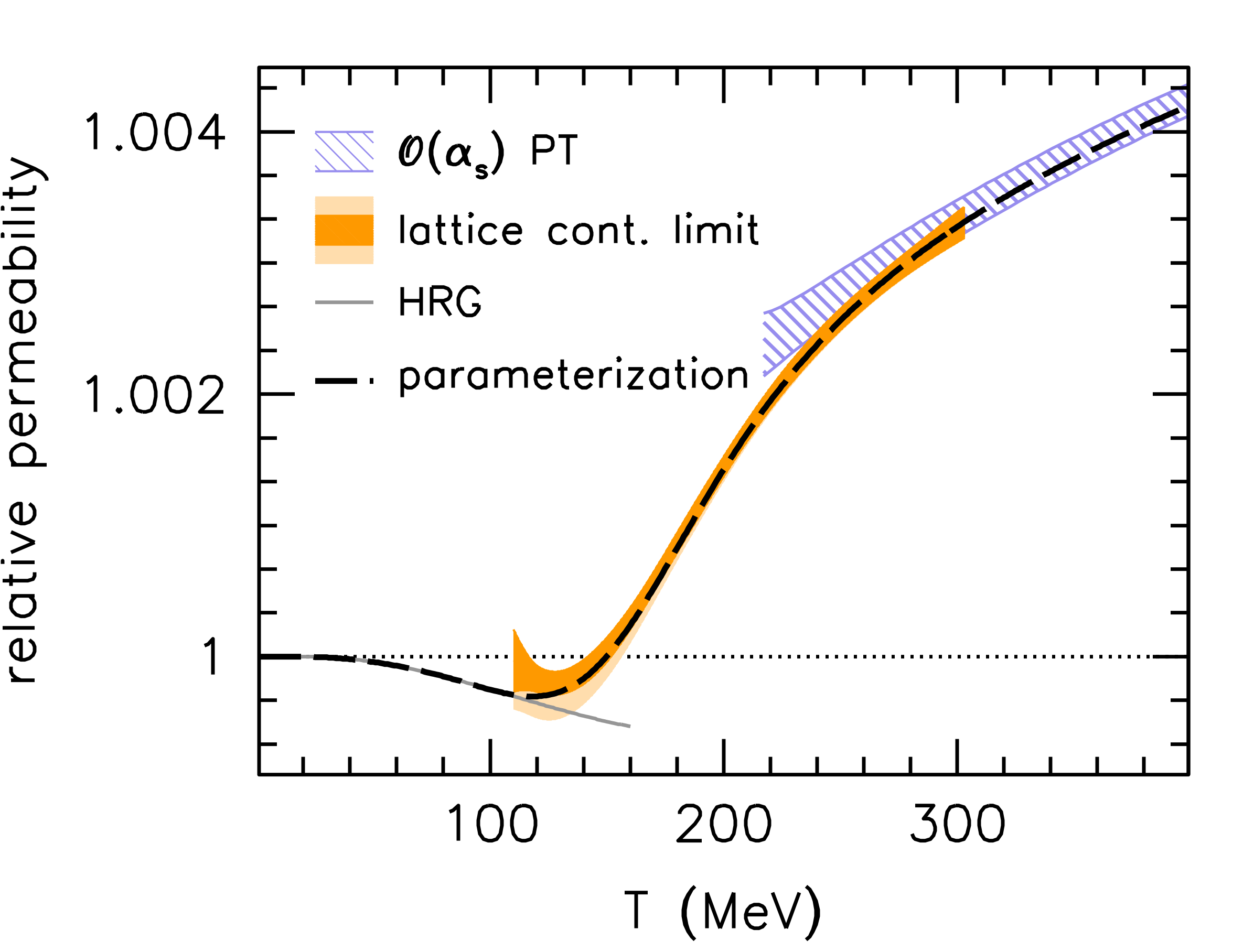The early Universe may undergo pion condensation for large lepton asymmetry [link].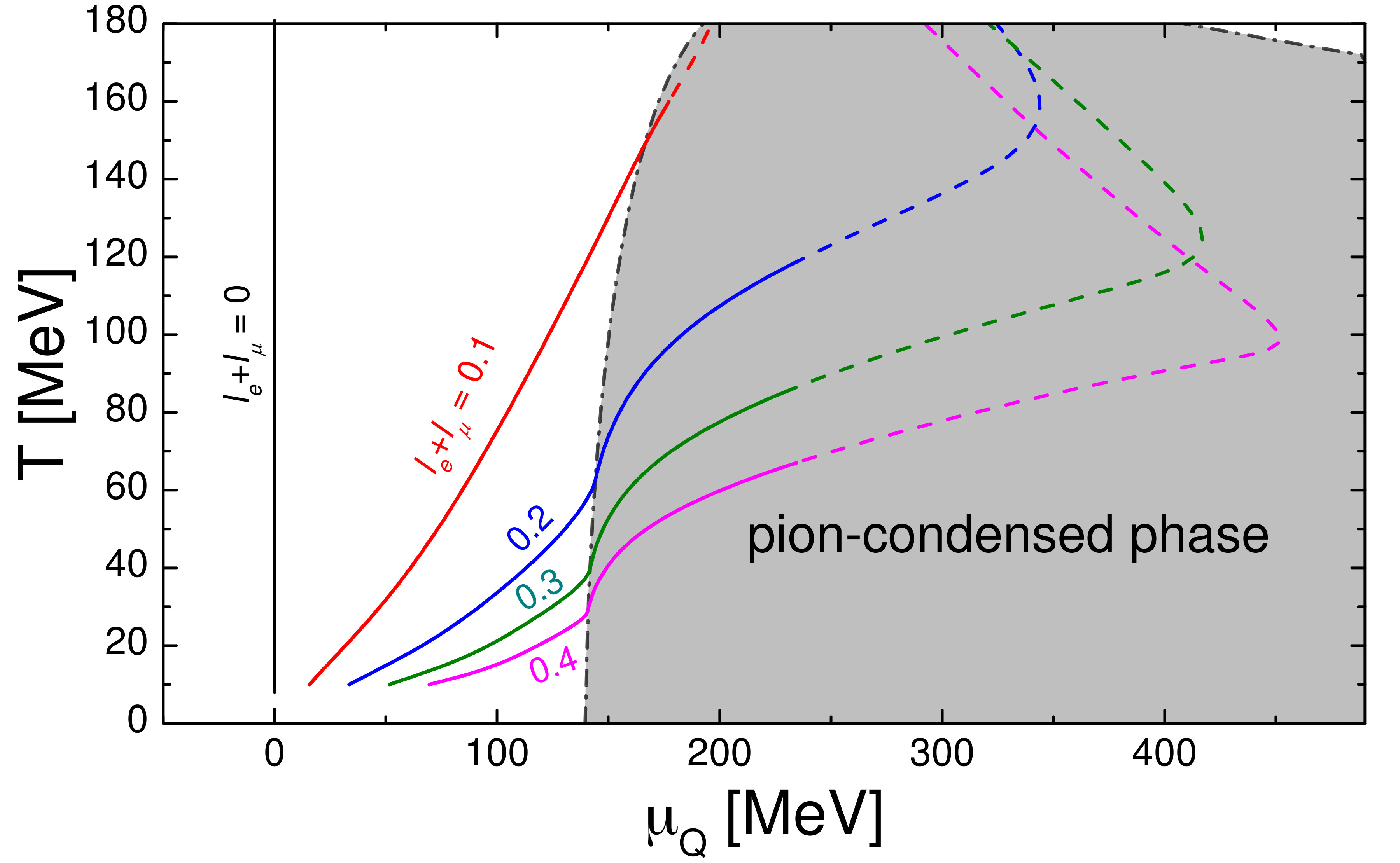The Dirac eigenvalues spread out in the complex plane as the isospin chemical potential grows. [link].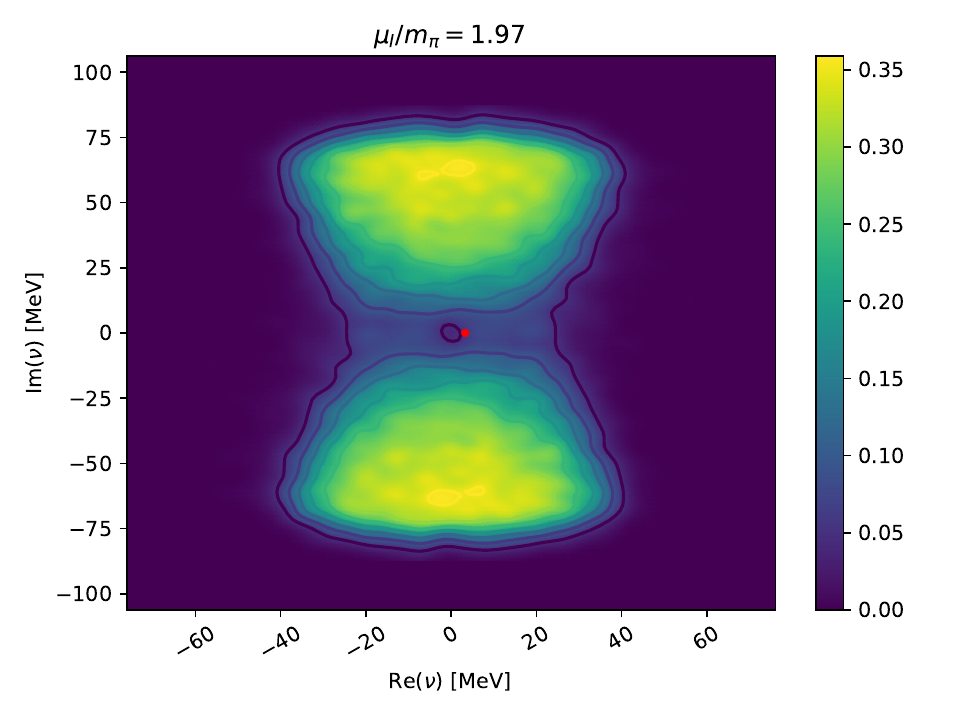A low-energy approximation of QCD is improved to match lattice results on inverse magnetic catalysis [link].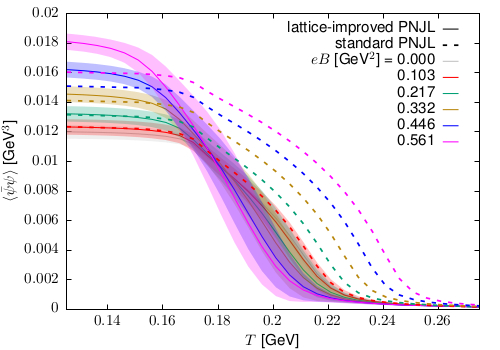Charged pions decay orders of magnitude faster if exposed to strong magnetic fields [link].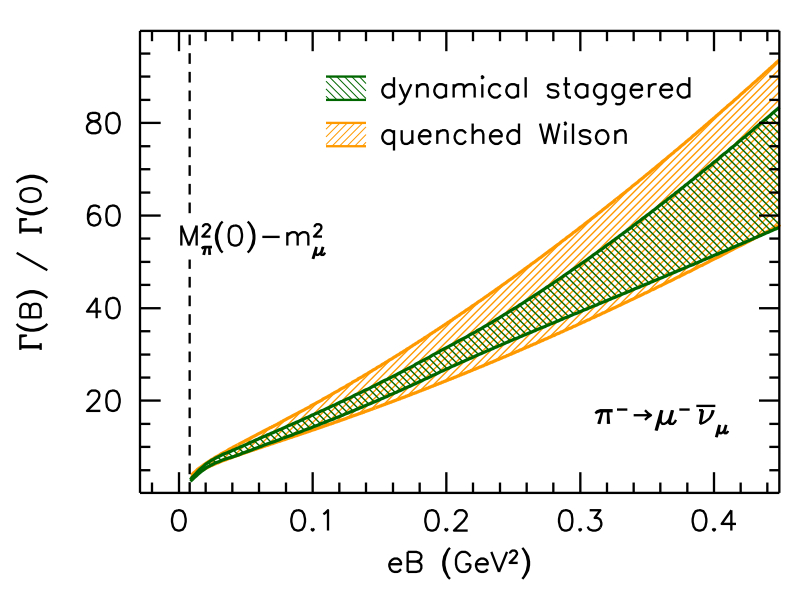The phase diagram of isospin-dense QCD exhibits a rich structure [link]. Figure represented in PRD's Kaleidoscope.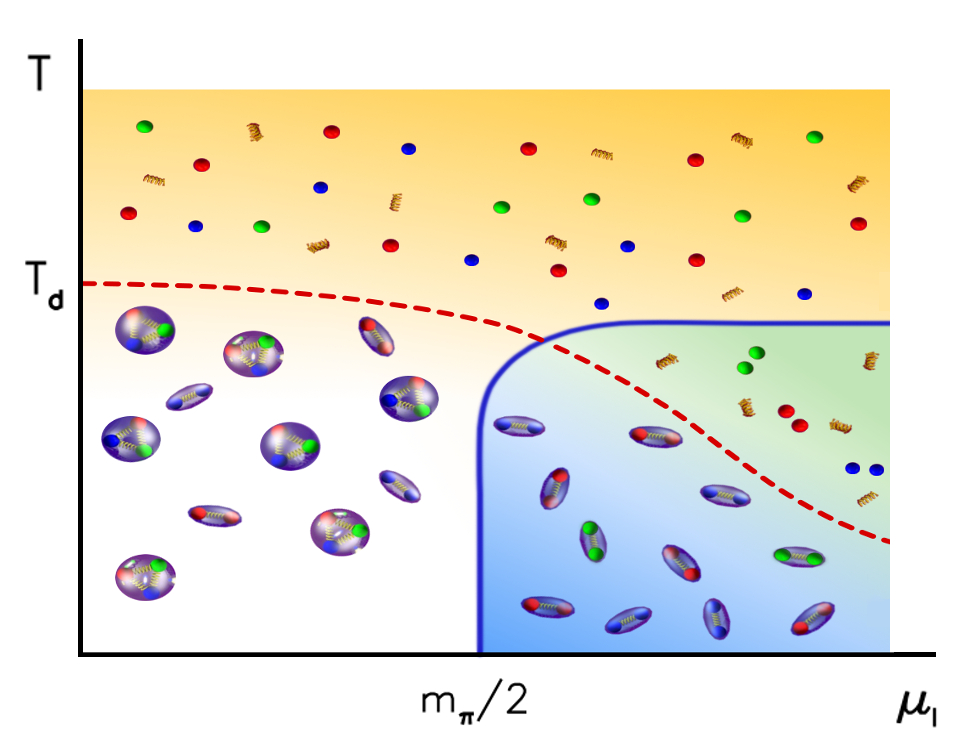A generative neural network learns essential features of lattice scalar field theory [link].